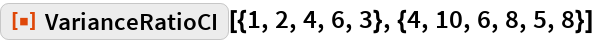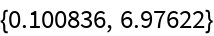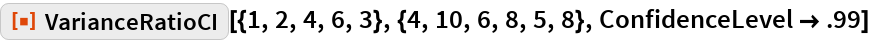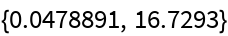#Function Repository Resource:

# VarianceRatioCI

Estimate the confidence interval of the ratio of the population variances

Contributed by: Wolfram Research
 ResourceFunction["VarianceRatioCI"][list1,list2] gives a confidence interval for the ratio of the population variances estimated from list1 and from list2.

## Details and Options

VarianceRatioCI[list1,list2] gives a confidence interval {min,max} for a ratio of the population variances estimated by Variance[list1] and Variance[list2].
For confidence level α, min=ratio/q(1+α)/2 and max=ratio/q(1-α)/2 where ratio=Variance[list1]/Variance[list2] and qi is the ith quantile of an F-ratio distribution with Length[list1]-1 numerator and Length[list2]-1 denominator degrees of freedom.
The following option can be given:
 ConfidenceLevel 0.95 probability associated with a confidence interval

## Examples

### Basic Examples

The 95% confidence interval for the ratio of two population variances:

 In:=Out=### Options

#### ConfidenceLevel

The 99% confidence interval:

 In:=Out=## Requirements

Wolfram Language 11.3 (March 2018) or above